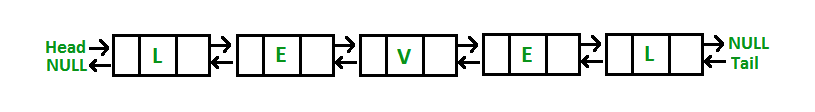Open In App

# Check if a doubly linked list of characters is palindrome or not

Given a doubly-linked list of characters, write a function that returns true if the given doubly linked list is a palindrome, else false.1. Create a doubly linked list where each node contains only one character of a string.
2. Initialize two pointers left at the start of the list and right at the end of the list.
3. Check if the data on the left node is equal to the right node. If it is equal, then increment left and decrement right till the middle of the list. If, at any stage, it is not equal, then return false.

Implementation:

## C++

 `// C++ program to check if doubly``// linked list is palindrome or not``#include``using` `namespace` `std;` `// Structure of node``struct` `Node``{``    ``char` `data;``    ``struct` `Node *next;``    ``struct` `Node *prev;``};` `/* Given a reference (pointer to pointer) to``   ``the head of a list and an int, inserts a``   ``new node on the front of the list. */``void` `push(``struct` `Node** head_ref, ``char` `new_data)``{``    ``struct` `Node* new_node = ``new` `Node;``    ``new_node->data  = new_data;``    ``new_node->next = (*head_ref);``    ``new_node->prev = NULL;``    ``if` `((*head_ref) !=  NULL)``      ``(*head_ref)->prev = new_node ;``    ``(*head_ref)    = new_node;``}` `// Function to check if list is palindrome or not``bool` `isPalindrome(``struct` `Node *left)``{``    ``if` `(left == NULL)``       ``return` `true``;` `    ``// Find rightmost node``    ``struct` `Node *right = left;``    ``while` `(right->next != NULL)``        ``right = right->next;` `    ``while` `(left != right)``    ``{``        ``if` `(left->data != right->data)``            ``return` `false``;``        ` `        ``if``(left->next == right)``          ``break``;``        ``left = left->next;``        ``right = right->prev;``    ``}` `    ``return` `true``;``}` `// Driver program``int` `main()``{``    ``struct` `Node* head = NULL;``    ``push(&head, ``'l'``);``    ``push(&head, ``'e'``);``    ``push(&head, ``'v'``);``    ``push(&head, ``'e'``);``    ``push(&head, ``'l'``);` `    ``if` `(isPalindrome(head))``        ``printf``(``"It is Palindrome"``);``    ``else``        ``printf``(``"Not Palindrome"``);` `    ``return` `0;``}`

## Java

 `// Java program to check if doubly``// linked list is palindrome or not``class` `GFG``{` `// Structure of node``static` `class` `Node``{``    ``char` `data;``    ``Node next;``    ``Node prev;``};` `/* Given a reference (pointer to pointer) to``the head of a list and an int, inserts a``new node on the front of the list. */``static` `Node push(Node head_ref, ``char` `new_data)``{``    ``Node new_node = ``new` `Node();``    ``new_node.data = new_data;``    ``new_node.next = head_ref;``    ``new_node.prev = ``null``;``    ``if` `(head_ref != ``null``)``    ``head_ref.prev = new_node ;``    ``head_ref = new_node;``    ``return` `head_ref;``}` `// Function to check if list is palindrome or not``static` `boolean` `isPalindrome(Node left)``{``    ``if` `(left == ``null``)``    ``return` `true``;` `    ``// Find rightmost node``    ``Node right = left;``    ``while` `(right.next != ``null``)``        ``right = right.next;` `    ``while` `(left != right)``    ``{``        ``if` `(left.data != right.data)``            ``return` `false``;` `        ``left = left.next;``        ``right = right.prev;``    ``}` `    ``return` `true``;``}` `// Driver program``public` `static` `void` `main(String[] args)``{``    ``Node head = ``null``;``    ``head = push(head, ``'l'``);``    ``head = push(head, ``'e'``);``    ``head = push(head, ``'v'``);``    ``head = push(head, ``'e'``);``    ``head = push(head, ``'l'``);` `    ``if` `(isPalindrome(head))``        ``System.out.printf(``"It is Palindrome"``);``    ``else``        ``System.out.printf(``"Not Palindrome"``);``}``}` `// This code is contributed by Rajput-Ji`

## Python3

 `# Python3 program to check if doubly``# linked list is a palindrome or not` `class` `Node:`` ` `    ``def` `__init__(``self``, data, ``next``, prev):``        ``self``.data ``=` `data``        ``self``.``next` `=` `next``        ``self``.prev ``=` `prev` `# Given a reference (pointer to pointer) to``# the head of a list and an int, inserts``# a new node on the front of the list.``def` `push(head_ref, new_data):`` ` `    ``new_node ``=` `Node(new_data, head_ref, ``None``)` `    ``if` `head_ref !``=` `None``:``        ``head_ref.prev ``=` `new_node``        ``head_ref ``=` `new_node``        ` `    ``return` `head_ref`` ` `# Function to check if list is palindrome or not``def` `isPalindrome(left):`` ` `    ``if` `left ``=``=` `None``:``        ``return` `True` `    ``# Find rightmost node``    ``right ``=` `left``    ``while` `right.``next` `!``=` `None``:``        ``right ``=` `right.``next` `    ``while` `left !``=` `right:``     ` `        ``if` `left.data !``=` `right.data:``            ``return` `False` `        ``left ``=` `left.``next``        ``right ``=` `right.prev``     ` `    ``return` `True`` ` `# Driver program``if` `__name__ ``=``=` `"__main__"``:`` ` `    ``head ``=` `None``    ``head ``=` `push(head, ``'l'``)``    ``head ``=` `push(head, ``'e'``)``    ``head ``=` `push(head, ``'v'``)``    ``head ``=` `push(head, ``'e'``)``    ``head ``=` `push(head, ``'l'``)` `    ``if` `isPalindrome(head):``        ``print``(``"It is Palindrome"``)``    ``else``:``        ``print``(``"Not Palindrome"``)` `# This code is contributed by Rituraj Jain`

## C#

 `// C# program to check if doubly``// linked list is palindrome or not``using` `System;` `class` `GFG``{` `// Structure of node``public` `class` `Node``{``    ``public` `char` `data;``    ``public` `Node next;``    ``public` `Node prev;``};` `/* Given a reference (pointer to pointer) to``the head of a list and an int, inserts a``new node on the front of the list. */``static` `Node push(Node head_ref, ``char` `new_data)``{``    ``Node new_node = ``new` `Node();``    ``new_node.data = new_data;``    ``new_node.next = head_ref;``    ``new_node.prev = ``null``;``    ``if` `(head_ref != ``null``)``    ``head_ref.prev = new_node ;``    ``head_ref = new_node;``    ``return` `head_ref;``}` `// Function to check if list is palindrome or not``static` `bool` `isPalindrome(Node left)``{``    ``if` `(left == ``null``)``    ``return` `true``;` `    ``// Find rightmost node``    ``Node right = left;``    ``while` `(right.next != ``null``)``        ``right = right.next;` `    ``while` `(left != right)``    ``{``        ``if` `(left.data != right.data)``            ``return` `false``;` `        ``left = left.next;``        ``right = right.prev;``    ``}` `    ``return` `true``;``}` `// Driver program``public` `static` `void` `Main(String[] args)``{``    ``Node head = ``null``;``    ``head = push(head, ``'l'``);``    ``head = push(head, ``'e'``);``    ``head = push(head, ``'v'``);``    ``head = push(head, ``'e'``);``    ``head = push(head, ``'l'``);` `    ``if` `(isPalindrome(head))``        ``Console.Write(``"It is Palindrome"``);``    ``else``        ``Console.Write(``"Not Palindrome"``);``}``}` `// This code is contributed by Rajput-Ji`

## Javascript

 ``

Output

```It is Palindrome

```

Time complexity: O(n)
Auxiliary Space : O(1)

Related Post:

This article is contributed by Akash Gupta. If you like GeeksforGeeks and would like to contribute, you can also write an article using write.geeksforgeeks.org or mail your article to review-team@geeksforgeeks.org. See your article appearing on the GeeksforGeeks main page and help other Geeks.